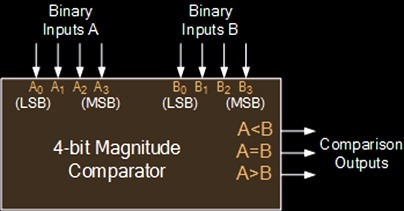# VHDL code for 4-bit binary comparator

Binary comparator compare two 4-bit binary number. It is also known as magnitude comparator and digital comparator. Analog form comparator is voltage comparator. The functionality of this comparator circuit is, It consist of 3 outputs Greater, Equal and Smaller. If inp-A is greater then inp-B then greater output is high, if both inp-A and inp-B are same then equal output is high, else smaller output is high.

VHDL Code for 4-bit Binary Comparator

```Library ieee;
use ieee.std_logic_1164.all;
use ieee.std_logic_arith.all;
use ieee.std_logic_unsigned.all;

entity VHDL_Binary_Comparator is
port (
inp-A,inp-B   : in std_logic_vector(3 downto 0);
greater, equal, smaller  : out std_logic
);
end VHDL_Binary_Comparator ;

architecture bhv of VHDL_Binary_Comparator is
begin
greater <= '1' when (inp-A > inp-B)
else '0';
equal <= '1' when (inp-A = inp-B)
else '0';
smaller <= '1' when (inp-A < inp-B)
else '0';
end bhv;```